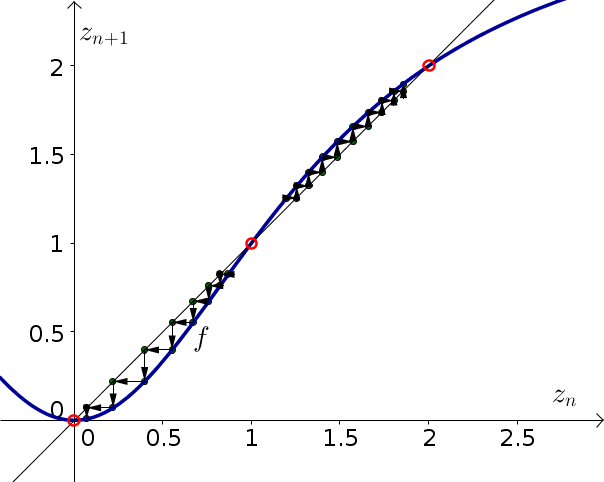# Math Insight

### Image: Discrete dynamical system example function 3, with cobwebbingThe function $z_{n+1}=f(z_n)=\frac{3z_n^2}{2+z_n^2}$ is plotted in blue along with the diagonal line $z_{n+1}=z_n$. Equilibria of the dynamical system are illustrated by the red circles at the points where the two graphs intersect. Cobwebbing is indicated by the arrows.

Source image file: discrete_dynamical_system_example_function_3_cobweb.ggb
Source image type: Geogebra